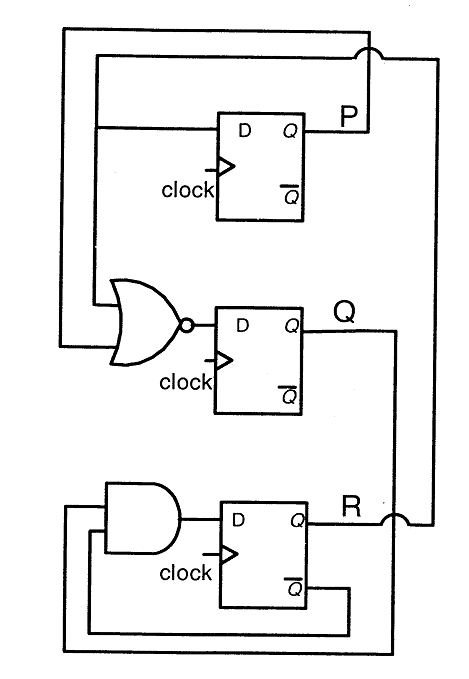# GATE | GATE CS 2011 | Question 65

Consider the following circuit involving three D-type flip-flops used in a certain type of counter configuration.If at some instance prior to the occurrence of the clock edge, P, Q and R have a value 0, 1 and 0 respectively, what shall be the value of PQR after the clock edge?
(A) 000
(B) 001
(C) 010
(D) 011

Explanation: P’ = R
Q’ = (P + R)’
R’ = QR’ Given that (P, Q, R) = (0, 1, 0), next state P’, Q’, R’ = 0, 1, 1

———————————————————————————————–

D flip flop truth table

 D Q(t+1) 0 0 1 1

Initially (p,q,r) =(0,1,0)
D for p=R
D for q=NOT(p xor r)
D for r= (not)r.q
So Q(t+1) for(p,q,r)
p=>r=0 so p=0
q=> NOT(p xor r) => 1      so q=1
r=>(not)r.q => 1         so r=1
(p, q, r) = (0, 1, 1)

Alternative approach –
Truth table of a D Flip-Flop-By looking at the circuit diagram, it is clear that the boolean expressions of P, Q, and R are-
Here the subscript t refers to the current clock cycle, and the subscript (t+1) refers to the next clock cycle.This explanation is provided by Chirag Manwani.

Quiz of this Question

My Personal Notes arrow_drop_up
Article Tags :

Be the First to upvote.

Please write to us at contribute@geeksforgeeks.org to report any issue with the above content.